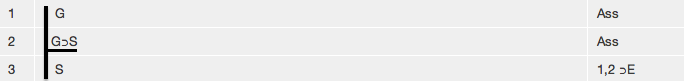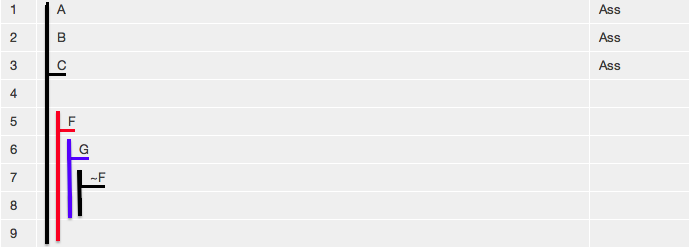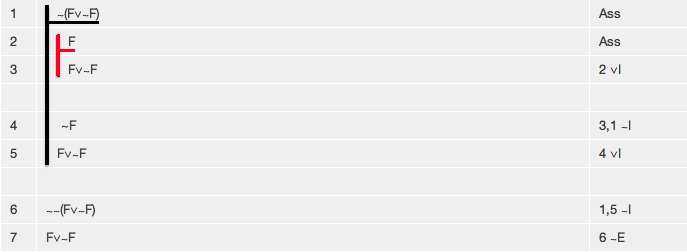# Tutorial 6: What is a derivation and what does it prove? How experts do derivations.

Logical System

2013

### Skills to be acquired in this tutorial:

a) Understanding the nature of derivation. b) Learning elementary Tactics.

### The Tutorial:

a)

A derivation or proof consists of a finite list of lines.

Each line in the list, if understood appropriately, represents something valid. In particular, the last line in the list depicts the argument or theorem under consideration and it is valid. So the derivation amounts to a proof of the validity of the argument or of a theorem.

For example, the argument

If Arthur is wearing a green tie, he will stand out in a crowd.
Arthur is wearing a green tie.

Therefore,

Arthur stands out in a crowd.

symbolized to

G⊃S,
G

S

has the following proof or derivationEach line carries with it three items of information. There is the formula that is justified or supported by the line, in this case the formula S. There is the justification of the line, in this case 1,2 ⊃E (which tells us that this line was obtained from lines 1 and 2 by an application of the rule ⊃E). The third item is a little more subtle. Let us digress for one moment. What does the above proof prove? Does it prove that S (that 'Arthur stands out in a crowd')? Surely not, the above argument is valid, the proof is correct, yet for all this it might be that S is false(that, in fact, 'Arthur does not stand out in a crowd'). What we have proved is that S, assuming that G⊃S and G. Each line tells of its own assumptions. The vertical line between the line number 3 and the S is the notation that does this.

Easy Deriver uses a vertical line notation to show which assumptions are current. There are the premises or standing assumptions which run through the entire derivation. These are depicted by being written in a list against a vertical line with a horizontal line under the last one. The vertical line then continues as long as the assumptions apply, thus:In the derivation below, this signifies that A,B,C are assumptions of all lines marked by their vertical line, 1 to 8 inclusive in this case. The notation is also extended beyond the standing assumptions to other assumptions which may be present for a while:This time A,B,C are assumptions of lines 1 to 9 inclusive. F of 5,6,7,8,9. G of 6,7, 8. ∼F of 7 and 8.

(As a quick exercise, list the assumptions of lines 1,3,6,7 in the following derivationAnswer: line 1 has assumption ∼(F∨∼F), line 3 has assumptions ∼(F∨∼F) and F, line 6 has no assumptions (the empty set of assumptions) and line 7 also has no assumptions.)

Some arguments have no premises, and some theorems have no assumptions, so within Deriver there can be zero, one, or many standing assumptions and these are added all at once at the beginning of the derivation. Extra assumptions can be added during the course of a derivation; if so, they are added one at a time.

It is perfectly possible for a line to have no assumptions; in these cases we still associate a set of assumptions with the line, but, of course, that set is empty.

So, looking at the matter abstractly, each line in a derivation has the form

<line number> <assumptions> <formula> <justification>

and a derivation is a list of such lines, for example

1. <assumptions1> <formula1> <justification1>
2. <assumptions2> <formula2> <justification2>
3. <assumptions3> <formula3> <justification3>
4. <assumptions4> <formula4> <justification4> .

The <assumptions> aspect of a line is to some degree concealed and has to be accessed by unravelling the vertical line notation.

#### To review.

A derivation or proof consists of a finite sequence or list of lines. Each line consists of three components: its set of assumptions, its formula that is justified (given those assumptions), and its justification. Each line in a derivation is proved in its own right, but the focus of interest is usually on the last line in the list and this is considered to be the theorem proved.

There is no exercise to accompany the first part of this tutorial.

b)

What is often mysterious is how skilled logicians manage to do difficult derivations. Fortunately something useful can be said about this. Heuristics-- the study of how to solve problems-- has a history that is thousands of years old. Heuristics, in its modern guise, in this context, is what we will call Tactics.

Tactics are a simple yet extremely powerful problem solving heuristic. Further, Deriver is able to display and run Tactics in an effortless and unobstrusive way.

The idea behind Tactics is as follows. Say we are trying to prove A∧B,C ∴ B∧C. We think of the premise or premises, assumptions, and lines that we have proved so far, as the facts that we have, and the conclusion as the problem that we are trying to solve. There may be facts which are compound propositions containing connectives; in which case we can use the tactic of employing logical Rules which remove connectives to extend the facts. In the example, A∧B is among the facts and the Rule ∧E can be applied to this to extend the facts to A∧B, C, A, B. Turning now to the problem. It may be a compound formula containing a connective; in which case we can use the tactic of employing logical Rules which introduce connectives to anticipate sub-problems which have to be solved before the final problem. In the example B∧C is a compound proposition and we can guess that the Rule ∧I, applied to B and C separately, was used to obtain it; and thus the problem B∧C is removed and B and C become subproblems to be solved. A subproblem is solved by being or becoming one of the facts. And a proof is complete when there are no further problems or subproblems.

Deriver displays and implements all fact extending tactics with little or no difference from the standard production of proofs. You select the line or lines you wish to apply the Tactic to, and click the appropriate elimination Rule. For example, to extend the facts A∧B, C∧A, B you select a conjunction (the first one or the second one) and click on ∧E.

Deriver displays and implements all problem reducing tactics with one large difference from the standard production of proofs. Since there is only one conclusion or only one problem under consideration at a time, it is unique. So there is no point in selecting it, and there is no need for you to do so. All you do is to click the appropriate connective introducing Rule. For example, with the conclusion B∧C click ∧I. When a tactic is used to reduce a problem to more than one sub-problem, the question arises as to which sub-problem to consider first (since always only one problem is under consideration at one time). The usual approach here is to have a default. One can always look at the last available sub-problem first (and do a proof backwards). Or one can look at the first available sub-problem first (and do a proof forwards). Deriver allows you to change problems (merely by clicking on the appropriate question mark), so you are free to do as you wish. But, if there is no intervention from the User, Deriver will do proofs forwards.

## Exercises to accompany Tutorial 6

Just an observation about the program's ability to produce proofs under the Next Line and Derive It menu commands:- Finding a proof requires 'intelligence', and the program uses intelligence to find proofs. But then, as a theoretical fact, if an argument has one proof it will have infinitely many different proofs (some longer some shorter some more elegant some less elegant). Finding a short elegant proof, for any particular argument, adds a second level of 'intelligence'. The program does what it can here, but it cannot be sure to find the shortest most elegant proof for every valid argument (and nor can any human do this). What is of primary importance is the first step, finding a proof at all. The second step, finding a 'good' proof is not so important. We are using proofs as a means of determining whether arguments are valid; and that determination is settled entirely by the first step: finding a proof, any proof.

### Exercise 1 (of 3)

Each of the derivations in the following Exercise can be done using the Rule of Elimination of the Conditional ⊃E, and the rules learned previously.

If a formula in a line of a derivation has the form

<formula1>

and a formula in a line of a derivation has the form

<formula1> ⊃ <formula2>

the Rule of Elimination of the Conditional ⊃E allows you to add

<formula2>

The elimination of ⊃ {⊃E} is the classical inference rule known as Modus Ponens. In English, if you have A and If A then B you are entitled to B.

Show each of the following arguments to be valid.

(a) F, F⊃G ∴ G
(b) F⊃G, F ∴ G∧F
(c) F⊃G, G⊃H,F ∴H
(d) F⊃(G⊃H), F⊃G,F ∴H∧F

(e) F⊃((~G)⊃H), F⊃~G,F ∴H∧F

### Exercise 2 (of 3)

Each of the derivations in the following Exercise can be done using only the rule the Rule of Elimination of the Biconditional ≡E, and the rules learned previously.

If the formula in a line in a derivation has the form

<formula1>≡<formula2>

the Rule of Elimination of the Biconditional ≡E allows you to remove the biconditional and add either

<formula1>⊃<formula2>

or

<formula2>⊃<formula1>

as an extra line. You have a choice here (on the direction of the conditional).

Show each of the following arguments to be valid.

(a) F≡G ∴ G⊃F
(b) F≡G ∴ F⊃G
(c) F≡G, G ∴ H∨F
(d) F≡G, G≡H, F ∴ H

### Exercise 3 (of 3).

After you have started the proof switch on 'Tactics' under the Proof Wizard menu, and do the derivations in the following way. If any of the lines you wish to obtain has a connective in it, click the appropriate Introduction rule (in these examples this will split the problem in two). If any of the lines you have obtained has a connective in it, use an Elimination rule to take it out.

(a) F≡G, H∧G ∴G∧F

(b) (F∧H)∧(R∧G) ∴F∧G

If you decide to use the web application for the exercises you can launch it from here Deriver [Gentzen] — username 'logic' password 'logic'. Then either copy and paste the above formulas into the Journal or use the Deriver File Menu to Open Web Page with this address https://softoption.us/test/easyDeriver/CombinedExercisesEasyDGentzen.html .

Preferences

You may need to set some Preferences for this.

• you can check that the parser is set to gentzen.